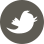# 论文阅读： AppProp: All-Pairs Appearance-Space Edit Propagation 核心内容翻译

9月 14, 2020 - 机器学习&大数据, 算法

## 概述

• 我们提出了一种精炼“粗糙”编辑的通用公式，该公式可确保将相似的编辑应用于外观相似的空间接近区域，并自然支持不同的艺术工作流程和用户界面。
• 我们基于矩阵采样得出了精确的近似算法，以交互方式传播最终编辑，并通过仔细的分析来支持我们的主张。
• 我们证明了我们的编辑框架可以处理多种外观量，包括低动态范围图像和高动态范围图像以及使用各种解析和非参数表示形式的测量材料
• 我们表明，我们的框架概括了先人基于笔画的图像和材料配方的工作，以及从联合双边过滤中得出的方法，同时在鲁棒性和效率方面提供了显着改进。

## 编辑框架

### 编辑传播

\sum_i \sum_j w_jz_{ij}(e_i-g_j)^2 + \lambda \sum_i \sum_j z_{ij}(e_i – e_j)^2

z_{ij} = e^{-\frac{|f_i-f_j|^2}{\sigma_a}} e^{-\frac{|x_i-x_j|^2}{\sigma_s}}

## 算法

(\sum_j z_{ij}w_j +2\lambda\sum_jz_{ij})e_i – 2\lambda\sum_{j}z_{ij}e_j = \sum_jz_{ij}w_jg_j

(D-Z)e = \frac{1}{2\lambda}ZWg
e和g是传播和初始化编辑的向量，Z是亲和度矩阵，元素就是z_{ij}，W是对角矩阵，W_{ii} = w_iD是对角矩阵D_{ii} = d_i = \sum_{j}(z_{ij}+z_{ij}w_j/2\lambda).

（线性系统的意思就是一个方程组，每一个i对应一个方程，因此按照上述定义放进去，两边同时除2\lambda前面一项就是De，这也是设置成对角矩阵的原因，每次都只有一项，就是与i对应的哪一项，Ze是后面一项，对于给定的i，计算要牵扯到所有的e，因此变成矩阵形式就是Ze；等式右边和第二项类似，每次都要牵扯所有的e来计算，因此写成ZWg。）

### 近似解

#### 低阶随机逼近

U = \left[\begin{matrix} A\\B\end{matrix}\right] \Rightarrow Z = \left[\begin{matrix} A&B^T\\B&C\end{matrix}\right]

V = \left[\begin{matrix} X\\Y\end{matrix}\right] \Rightarrow Z = \left[\begin{matrix} X\\Y\end{matrix}\right] \left[\begin{matrix} X&X^T\end{matrix}\right] = \left[\begin{matrix} XX^T&XY^T\\YX^T &YY^T\end{matrix}\right]

C = YY^T=(YX^T)((X^T)^{-1}X^{-1})(XY^T) = BA^{-1}B^T

Z \approx \widetilde{Z} = \left[\begin{matrix} A&B^T\\B &BA^{-1}B^T\end{matrix}\right] = \left[\begin{matrix} A\\B\end{matrix}\right] A^{-1}\left[\begin{matrix} A&B^T\end{matrix}\right] = UA^{-1}U^T

e \approx \frac{1}{2\lambda}(D-\widetilde{Z})^{-1}\widetilde{Z}Wg

d = (\frac{1}{2\lambda}ZW + Z)1_n \approx (\frac{1}{2\lambda}UA^{-1}U^TW + UA^{-1}U^T)1_n

(D-UA^{-1}U^T)^{-1} = D^{-1}-D^{-1}U(-A+U^TD^{-1}U)^{-1}U^TD^{-1}

e \approx \frac{1}{2\lambda}(D^{-1} D^{-1}U(-A+U^TD^{-1}U)^{-1}U^TD^{-1}) (UA^{-1}U^T)Wg

### 《论文阅读： AppProp: All-Pairs Appearance-Space Edit Propagation 核心内容翻译》有1个想法522上议院